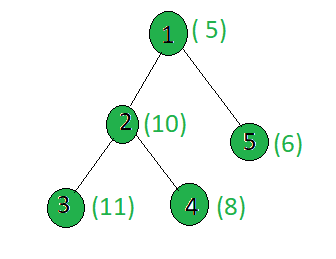# Count of all prime weight nodes between given nodes in the given Tree

Given a weighted tree containing N nodes, and two nodes u and v, the task is to find the count of nodes having prime weight on the simple path between u and v (both inclusive).

Examples:

Input:u = 3, v = 5
Output: 2
Explanation:
Prime weight on path 3 to 5 is [11, 5]. Hence the answer is 2.

Approach: To solve th problem mentioned above, the idea is to use the basic concept when we find the LCA of two nodes.

• Precompute all the prime numbers till MAX using Seive method to check if a number is prime or not in O(1)
• Given two nodes u and v, we will make both nodes at the same level, by moving the greater level node move upwards. As we move up we will also check if the weight is prime or not.
• If v == u then we will simply check the weight of current node and return the count.
• If v is not equal to u then we will move both u and v upward by 1 till they are not same.
• Now we will finally check the weight of the first ancestor of u or v and return the count.

Below is the implementation of the above approach:

 `// C++ program Count prime weight ` `// nodes between two nodes in the given tree ` ` `  `#include ` `using` `namespace` `std; ` `#define MAX 1000 ` ` `  `int` `weight[MAX]; ` `int` `level[MAX]; ` `int` `par[MAX]; ` `bool` `prime[MAX + 1]; ` `vector<``int``> graph[MAX]; ` ` `  `// Function to perform ` `// Sieve Of Eratosthenes for prime number ` `void` `SieveOfEratosthenes() ` `{ ` `    ``// Initialize all entries of prime it as true ` `    ``// A value in prime[i] will finally be false ` `    ``// if i is Not a prime, else true. ` `    ``memset``(prime, ``true``, ``sizeof``(prime)); ` ` `  `    ``for` `(``int` `p = 2; p * p <= MAX; p++) { ` ` `  `        ``// Check if prime[p] is not changed, ` `        ``// then it is a prime ` `        ``if` `(prime[p] == ``true``) { ` ` `  `            ``// Update all multiples ` `            ``// of p greater than or ` `            ``// equal to the square of it ` `            ``// numbers which are multiple ` `            ``// of p and are less than p^2 ` `            ``// are already been marked. ` `            ``for` `(``int` `i = p * p; i <= MAX; i += p) ` `                ``prime[i] = ``false``; ` `        ``} ` `    ``} ` `} ` ` `  `// Function to perform dfs ` `void` `dfs(``int` `node, ``int` `parent, ``int` `h) ` `{ ` `    ``// Stores parent of each node ` `    ``par[node] = parent; ` ` `  `    ``// Stores level of each node from root ` `    ``level[node] = h; ` ` `  `    ``for` `(``int` `child : graph[node]) { ` `        ``if` `(child == parent) ` `            ``continue``; ` `        ``dfs(child, node, h + 1); ` `    ``} ` `} ` ` `  `// Function to perform prime ` `// number between the path ` `int` `findPrimeOnPath(``int` `u, ``int` `v) ` `{ ` `    ``int` `count = 0; ` ` `  `    ``// The node which is present farthest ` `    ``// from the root node is taken as v ` `    ``// If u is farther from root node ` `    ``// then swap the two ` `    ``if` `(level[u] > level[v]) ` `        ``swap(u, v); ` ` `  `    ``int` `d = level[v] - level[u]; ` ` `  `    ``// Find the ancestor of v ` `    ``// which is at same level as u ` `    ``while` `(d--) { ` ` `  `        ``// If Weight is prime ` `        ``// increment count ` `        ``if` `(prime[weight[v]]) ` `            ``count++; ` ` `  `        ``v = par[v]; ` `    ``} ` ` `  `    ``// If u is the ancestor of v ` `    ``// then u is the LCA of u and v ` `    ``// Now check if weigh[v] ` `    ``// is prime or not ` `    ``if` `(v == u) { ` `        ``if` `(prime[weight[v]]) ` `            ``count++; ` `        ``return` `count; ` `    ``} ` ` `  `    ``// When v and u are on the same level but ` `    ``// are in different subtree. Now move both ` `    ``// u and v up by 1 till they are not same ` `    ``while` `(v != u) { ` `        ``if` `(prime[weight[v]]) ` `            ``count++; ` ` `  `        ``if` `(prime[weight[u]]) ` `            ``count++; ` ` `  `        ``u = par[u]; ` `        ``v = par[v]; ` `    ``} ` `    ``// If weight of first ancestor ` `    ``// is prime ` `    ``if` `(prime[weight[v]]) ` `        ``count++; ` ` `  `    ``return` `count; ` `} ` ` `  `// Driver code ` `int` `main() ` `{ ` `    ``// Precompute all the prime ` `    ``// numbers till MAX ` `    ``SieveOfEratosthenes(); ` ` `  `    ``// Weights of the node ` `    ``weight = 5; ` `    ``weight = 10; ` `    ``weight = 11; ` `    ``weight = 8; ` `    ``weight = 6; ` ` `  `    ``// Edges of the tree ` `    ``graph.push_back(2); ` `    ``graph.push_back(3); ` `    ``graph.push_back(4); ` `    ``graph.push_back(5); ` ` `  `    ``dfs(1, -1, 0); ` `    ``int` `u = 3, v = 5; ` `    ``cout << findPrimeOnPath(u, v) ` `         ``<< endl; ` ` `  `    ``return` `0; ` `} `

Output:

```2
```

Time Complexity: O(N)

Don’t stop now and take your learning to the next level. Learn all the important concepts of Data Structures and Algorithms with the help of the most trusted course: DSA Self Paced. Become industry ready at a student-friendly price.

My Personal Notes arrow_drop_upCheck out this Author's contributed articles.

If you like GeeksforGeeks and would like to contribute, you can also write an article using contribute.geeksforgeeks.org or mail your article to contribute@geeksforgeeks.org. See your article appearing on the GeeksforGeeks main page and help other Geeks.

Please Improve this article if you find anything incorrect by clicking on the "Improve Article" button below.